# AQA A Level Maths: Statistics复习笔记2.4.1 Correlation & Regression

### Scatter Diagrams

#### What does bivariate data mean?

• A lot of statistics is about looking at how different factors, or variables change how data behaves
• Bivariate data is data which is collected on two variables and looks at how one of the factors affects the other
• Each data value from one variable will be paired with a data value from the other variable
• The two variables are often related, but do not have to be

#### What is a scatter diagram?

• A scatter diagram is a way of graphing bivariate data
• You may be asked to plot, or add to, a scatter diagram
•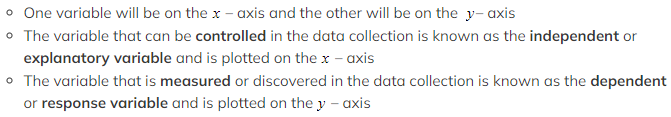Scatter diagrams allow statisticians to look for relationships between the two variables
• Some scatter diagrams will show a clear relationship know as correlation (see below)
• Others will not display on obvious relationship
• If a scatter diagram shows a relationship you may be asked to identify outliers

#### Worked Example

The scatter diagram below shows the number of Save My Exams question packs completed by a group of students and the percentage score they received in their A-Level Statistics exam.(i)State which of the variables is the explanatory variable and which is the response variable.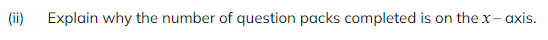(iii)Another student completed 50 question packs and scored 80% on their A Level Statistics exam, add this data to the scatter diagram.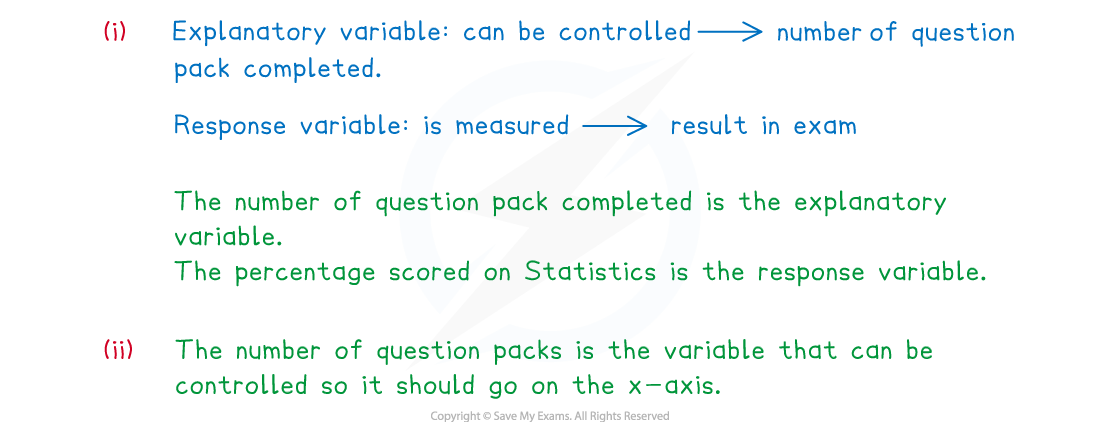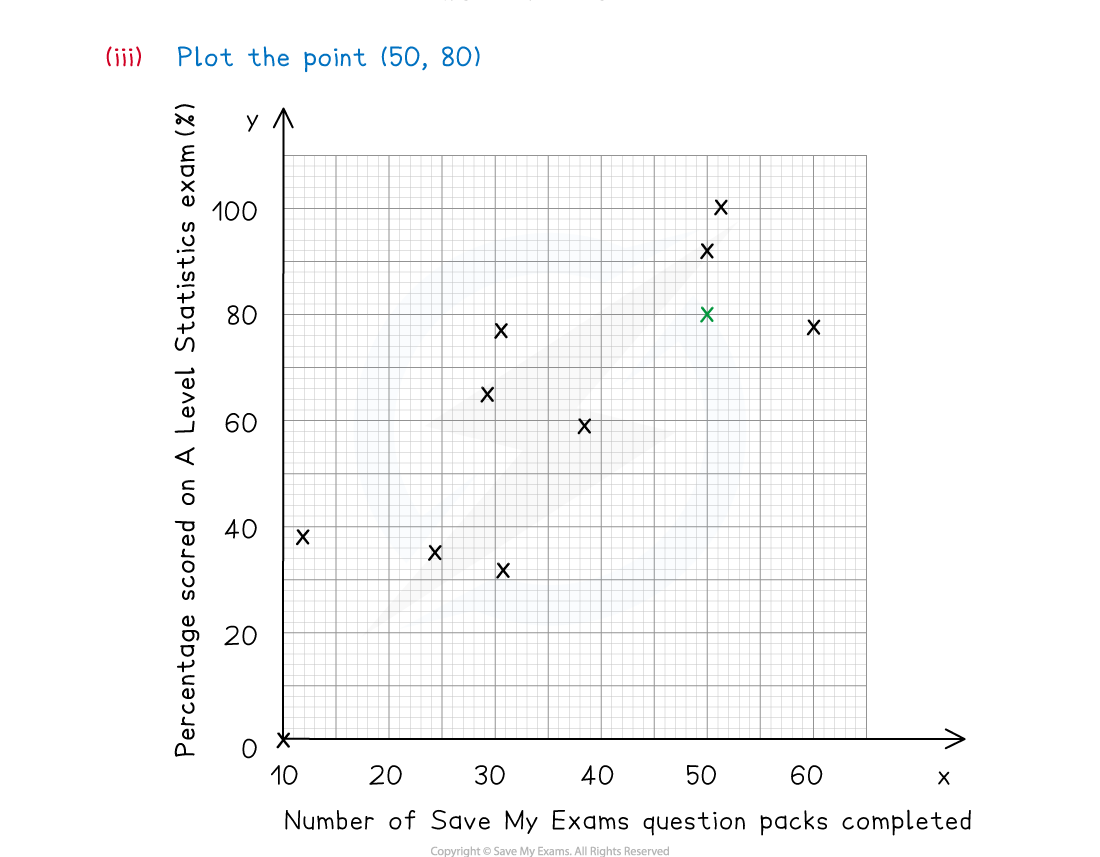#### Exam Tip

• Learn the vocabulary for the types of variables as you could be asked a question on this. Make sure you check the scales carefully when plotting any points.

### Correlation

#### What is correlation?

• Correlation is how the relationship between the two variables is described
• Perfect linear correlation means that the bivariate data will all lie on a straight line on a scatter diagram
• Linear correlation can be positive or negative and it can be strong or weak
• Positive correlation describes a data set where both variables are increasing
• Negative correlation describes a data set where one variable is increasing and the other is decreasing
• When describing correlation you should say whether it is positive or negative and also say whether it is strong or weak
• If correlation exists then there could be outliers, these will be data points that do not fit the pattern seen on the graph
• There will likely be a maximum of one or two outliers on any scatter diagram
• You may be asked to identify the outliers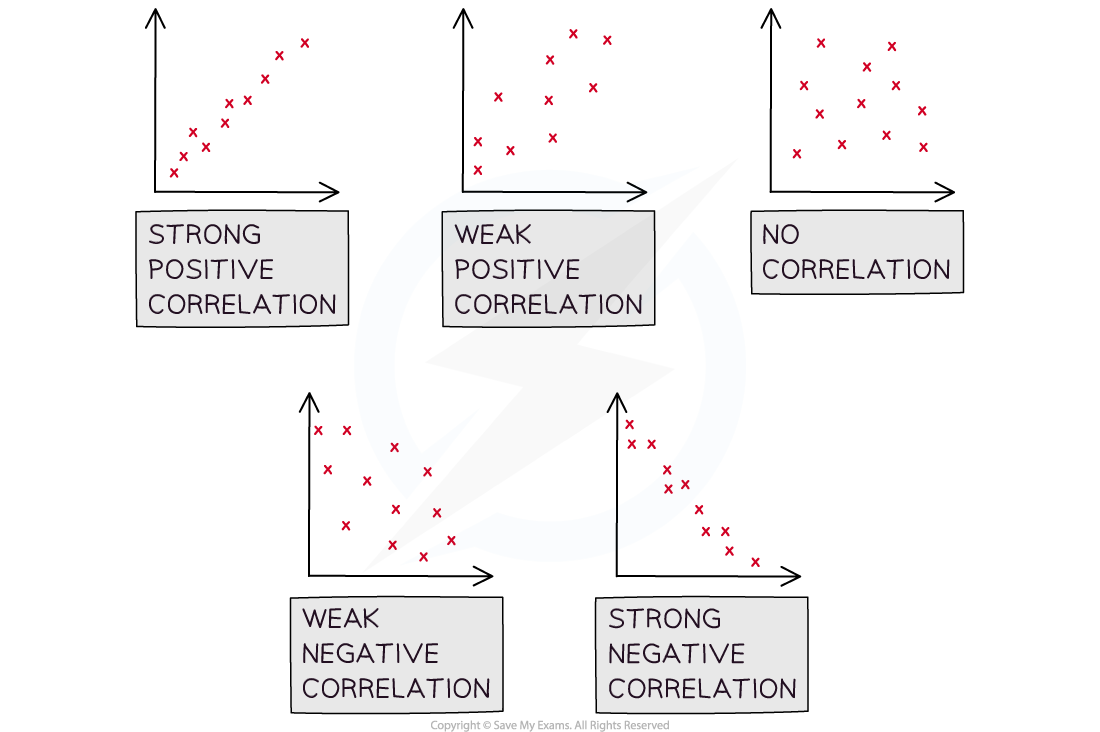#### What is the difference between correlation and causation?

• It is important to be aware that just because correlation exists, it does not mean that the change in one of the variables is causing the change in the other variable
• Correlation does not imply causation!
• If a change in one variable causes a change in the other then the two variables are said to have a causal relationship
• Observing correlation between two variables does not always mean that there is a causal relationship
• Look at the two variables in question and consider the context of the question to decide if there could be a causal relationship
• If the two variables are temperature and number of ice creams sold at a park then it is likely to be a causal relationship
• Correlation may exist between global temperatures and the number of monkeys kept as pets in the UK but they are unlikely to have a causal relationship
• Observing a relationship between two variables can allow you to create a hypothesis about those two variables

#### Worked Example

The scatter diagram below shows the number of Save My Exams question packs completed by a group of students and the percentage score they received in their A-Level Statistics exam.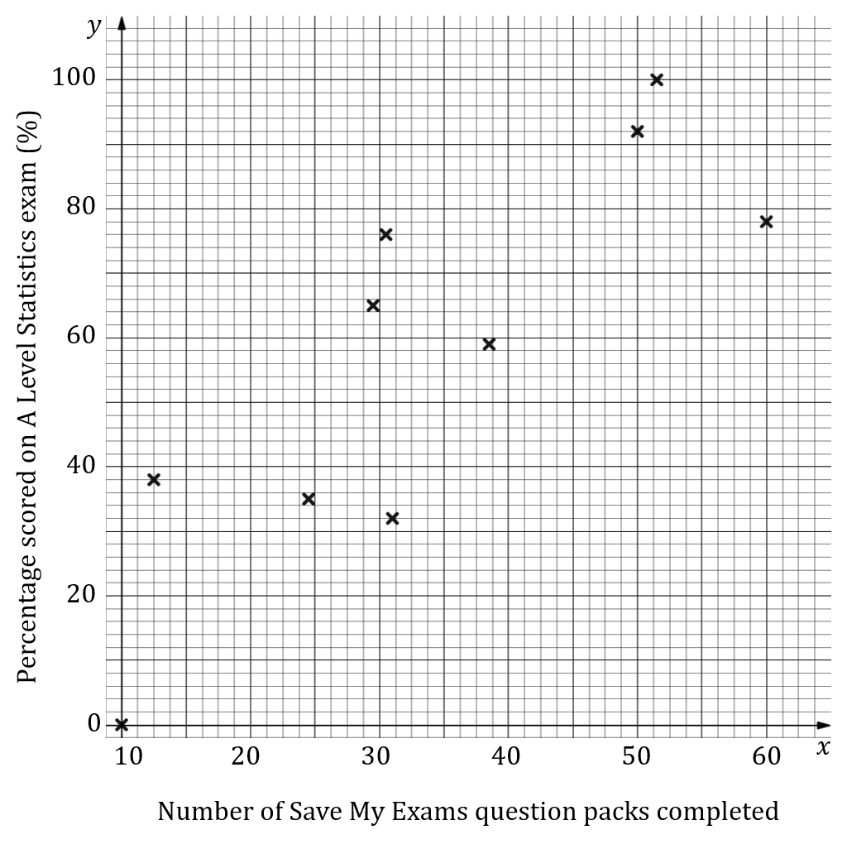(i)Describe the correlation shown in the scatter diagram.
(ii)Decide if you think there could be a causal relationship between the two variables and explain your reasoning.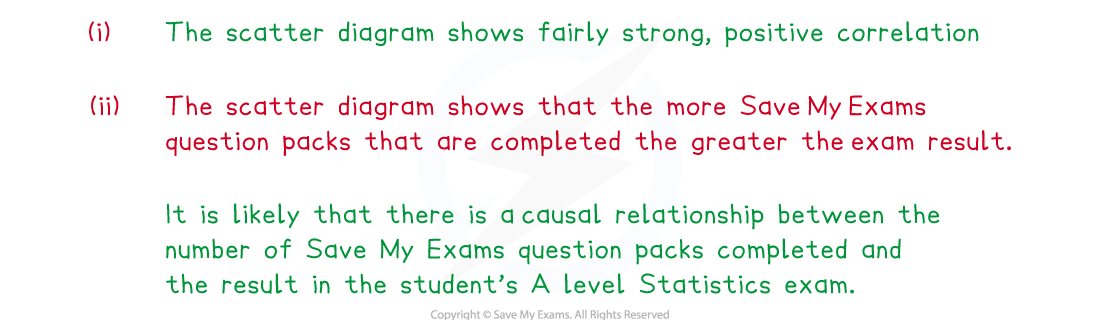### Linear Regression

#### What is linear regression?

• If strong linear correlation exists on a scatter diagram, then a line of best fit can be drawn
• This is a linear graph added to the scatter diagram that best approximates the relationship between the two variables
• At GCSE this will have been drawn by eye as a line that fits closest to the data values
• The data can be used to calculate the equation of the straight line that represents the best fit of the relationship between the two variables
• You do not need to know how to calculate it but you will need to be able to interpret one
• The least squares regression line is the line of best fit that minimises the sum of the squares of the gap between the line and each data value
• This is usually called the regression line and can be calculated either be looking at the vertical or the horizontal distances between the line and the data values
• If the regression line is calculated by looking at the vertical distances it is called the regression line of y on x
• If the regression line is calculated by looking at the horizontal distances it is called the regression line of x on y
• The regression line of x on y is rarely used and you are unlikely to come across it at this level

####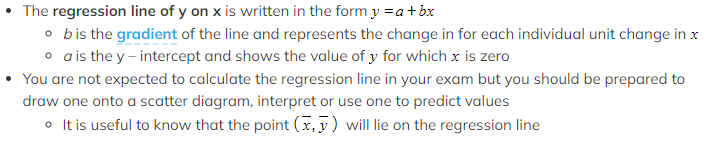How to use a regression line?

• Drawing a regression line is done in the same way as drawing a straight line graph, substitute some values from the independent data set to help you
• The regression line can be used to decide what type of correlation there is if there is no scatter diagram
• If b is positive then the data set has positive correlation and if b is negative then the data set has negative correlation
• The value of b can be used to interpret how the data is changing
•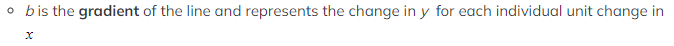The regression line can also be used to predict the value of a dependent variable from an independent variable
• Predictions should only be made for values of the dependent variable that are within the range of the given data
• Making a prediction within the range of the given data is called interpolation
• Making a prediction outside of the range of the given data is called extrapolation and is much less reliable
• The prediction will be more reliable if the number of data values in the original sample set is bigger

#### Worked Example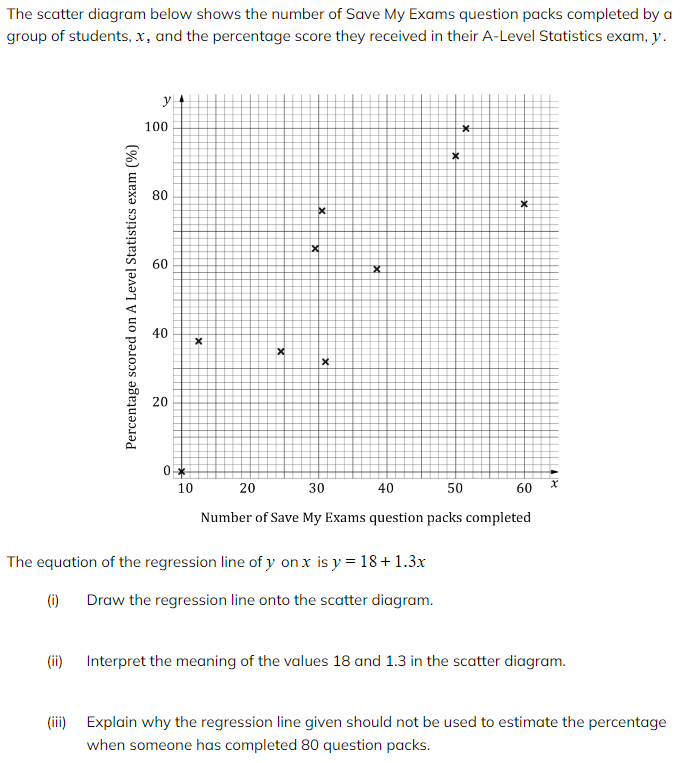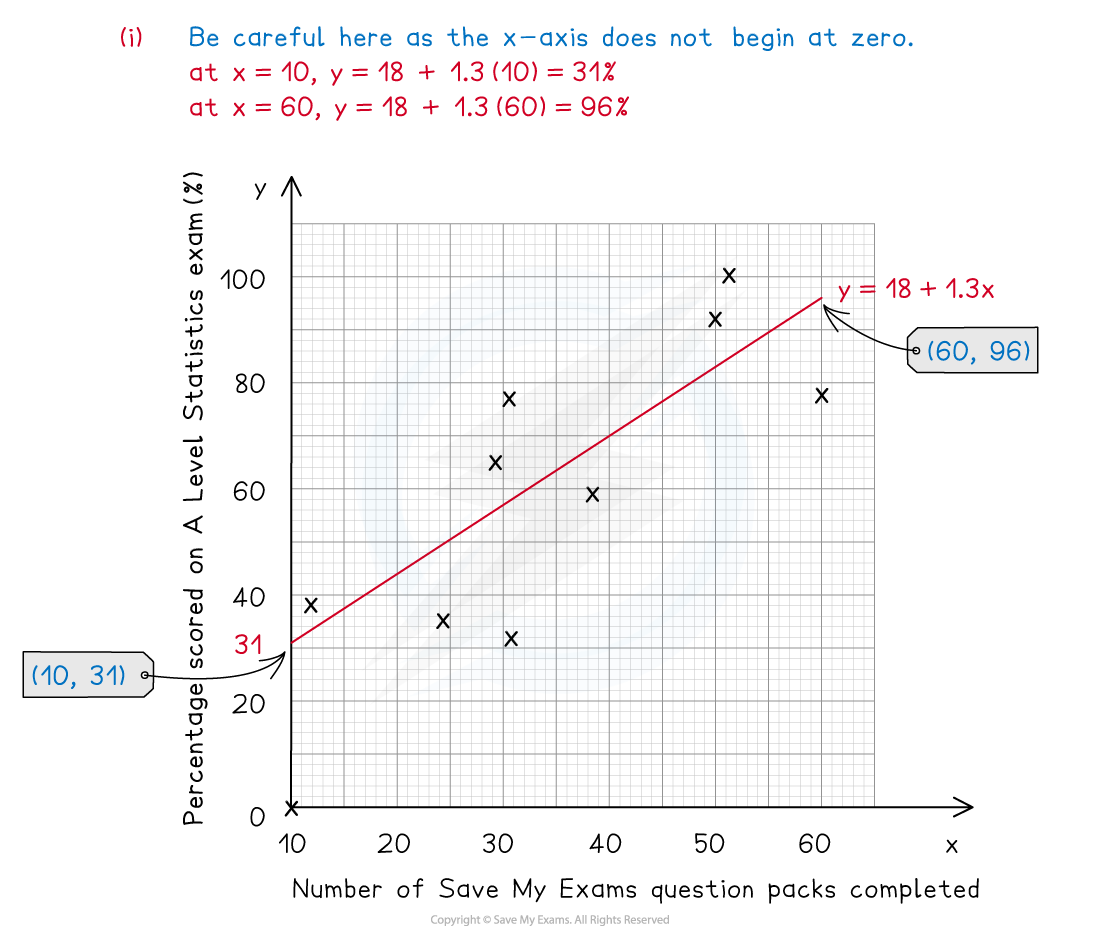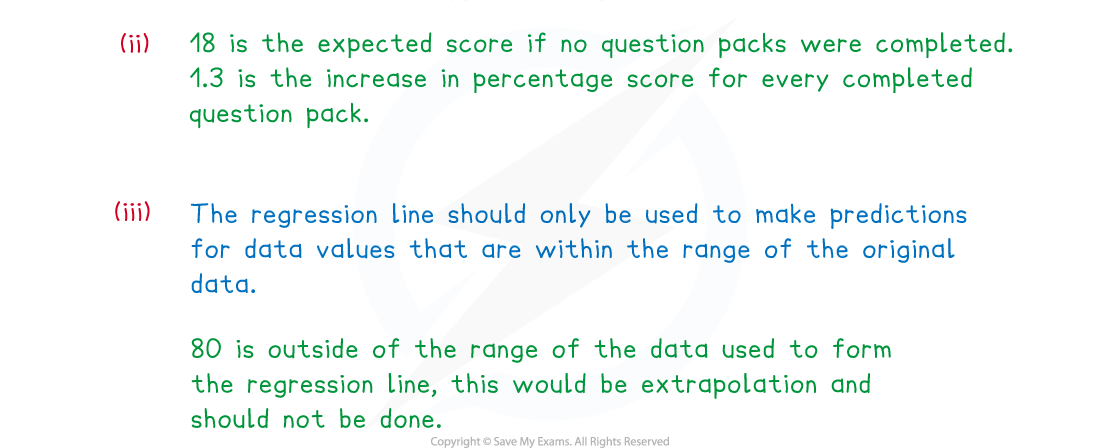#### Exam Tip

• Remember that the value of b is the gradient of the regression line, a greater value of b does not mean stronger correlation. When using a regression line to make a prediction make sure that the value you are predicting from falls within the range of the data used to calculate the regression line.